# Geometry of straight lines

You probably know exactly what is meant by a line. In this chapter, you will learn about line segments and rays and how they differ from lines. You will also learn more about parallel and perpendicular lines and how we indicate them on a diagram.

## Line segments, lines and rays

### Line segments

1. Measure each side of this quadrilateral. Write the measurements at each side.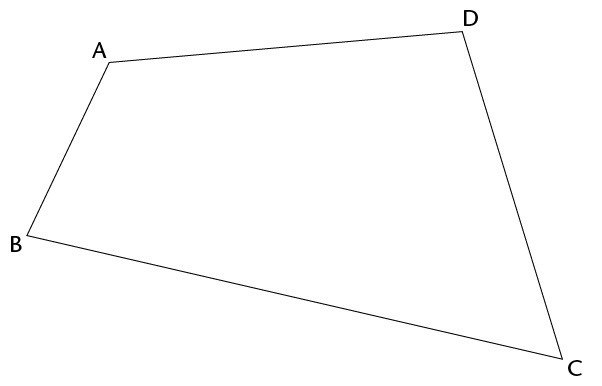Each side of a quadrilateral is a line segment.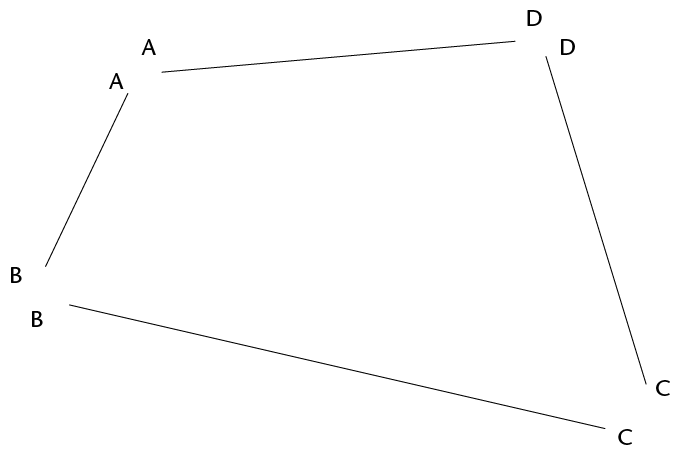A line segment has a definite starting point and a definite endpoint. We can draw and measure line segments.

2. Draw a line segment that is 12 cm long.

### Lines and rays

We can think of lines that have no ends, although we cannot draw them completely. We draw line segments to represent lines. When we draw a line segment to represent a line, we may put arrows at both ends to show that it goes on indefinitely on both sides.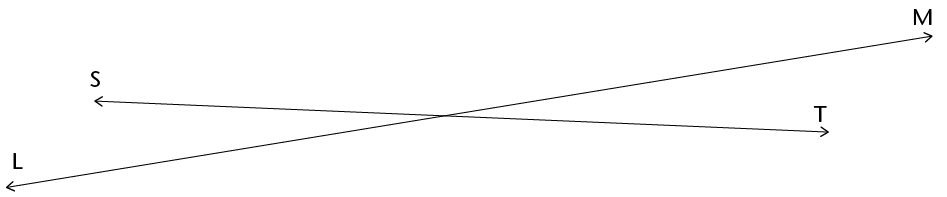The word line is used to indicate a line that goes on in both directions. We can only see and draw part of a line. A line cannot be measured.

1. Draw line AB.2. Did you draw the whole of line AB? Explain.

We can also think of a line that has a definite starting point but goes on indefinitely at the other end. This is called a half-line or a ray.

We can draw the starting point and part of a ray, using an arrow to indicate that it goes on at the one end.

Ray PQ goes on towards the right: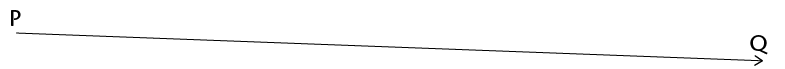Ray DC goes on towards the left: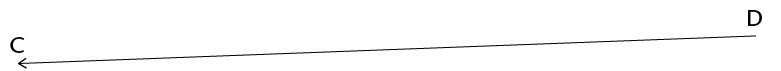3. Draw ray EF.4. Did you draw the whole of ray EF? Explain.

5. Do line segments XY and GH meet anywhere?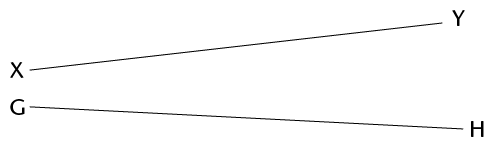6. Do lines KL and NP meet anywhere?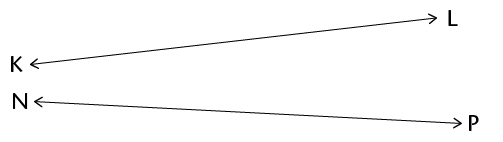7. Do rays AB and CD meet anywhere?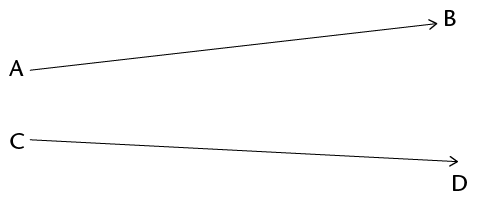8. Do rays FT and MW meet anywhere?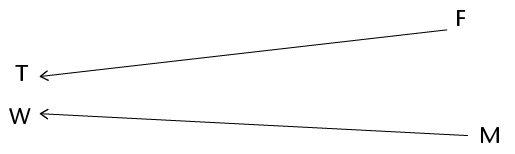9. Do rays JK and RS meet anywhere?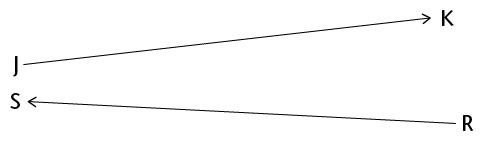## Parallel and perpendicular lines

### Parallel lines

Two lines that are a constant distance apart are called parallel lines. Lines AG and BH below are parallel. The symbol || is used to indicate parallel lines. We write: AG || BH.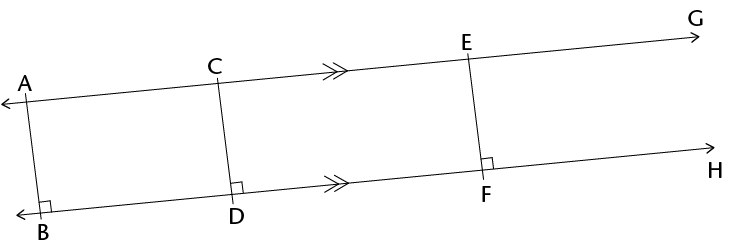1. Measure the distance between the two lines:

1. at A and B

2. at C and D

3. at E and F

Here are some more parallel lines: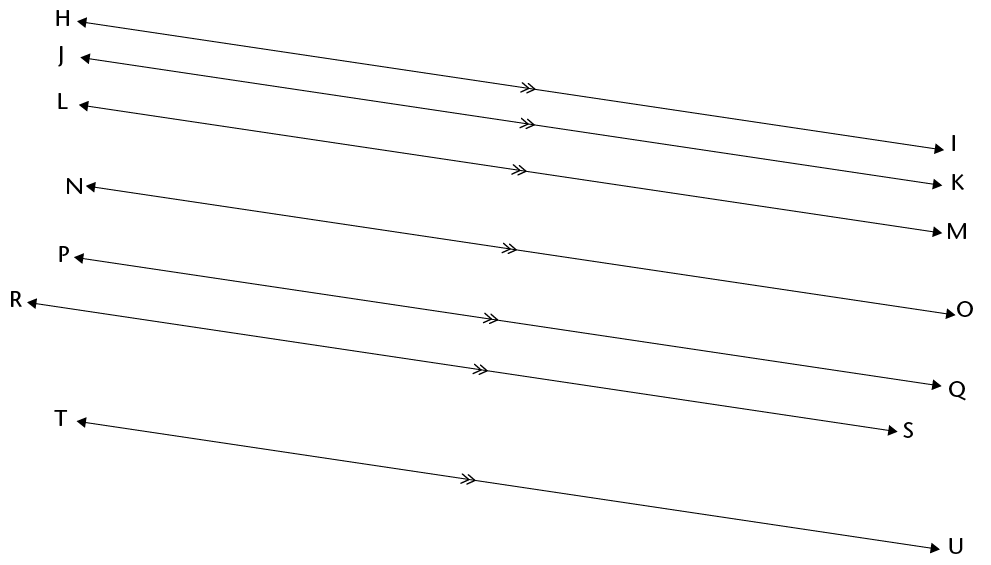2. Draw two parallel lines.

3. Draw three lines that are parallel to each other.

4. Will parallel lines meet somewhere?

5. Do you think lines PQ and ST are parallel? How can you check?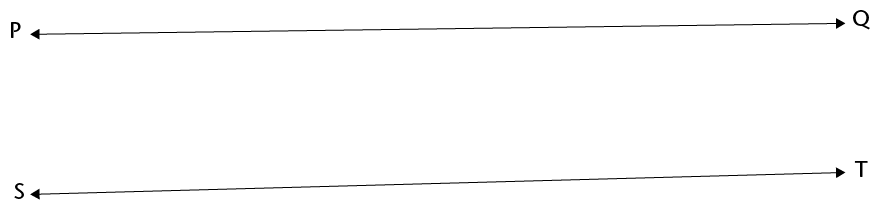1. Draw two lines that are almost parallel, but not quite.

2. Describe what you did to make sure that your two lines are not parallel.

6. Can two line segments be parallel?

7. Are line segments DK and FS parallel?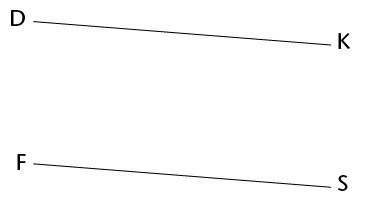8. Are line segments MN and AB parallel?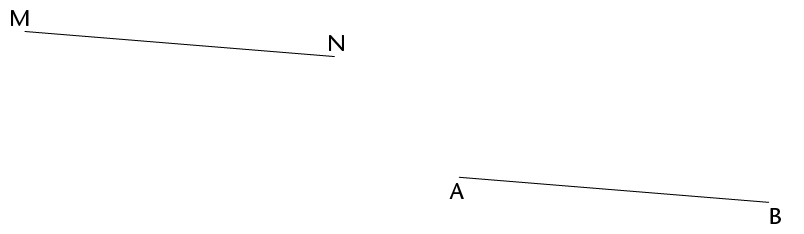9. What can you do so that you will be better able to check whether the above two line segments are parallel or not?

10. Can a line be parallel on its own?

11. Draw a line that is parallel to line XY above.

### Perpendicular lines

Lines CD and KL below are perpendicular to each other. The symbol is used to indicate perpendicular lines. We write: CD KL.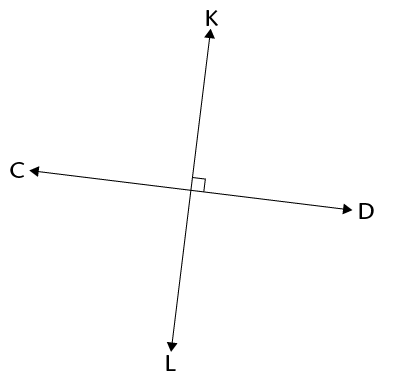1. How many angles are formed at the point where the above two lines meet?

Two lines that form right angles are perpendicular to each other.

2. Draw two rays that have the same starting point.3. Draw two rays that are perpendicular to each other and have the same starting point.4. Draw two rays that meet, but not at their starting points.5. Draw two rays that meet but not at their starting points, and that are perpendicular to each other.6. Can you draw two rays that have the same starting point, and are parallel to each other?